Neutral curve (1)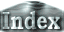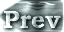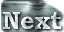We linearize the governing equations by assuming small amplitudes for y and q. Further, we expand y and q by a series of functions satisfying the boundary conditions and truncate up to the vertical wavenumber 1 (see Appendix B). As a result, we can easily obtain the approximate expression of the neutral curve of the system.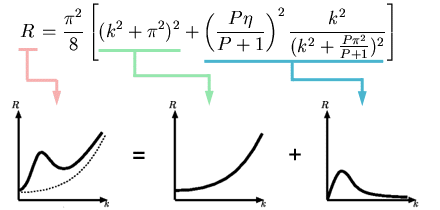(11)

The first term on the right hand side   (p2/8)(k2+p2)2 describes the neutral curve for the case of the non-rotating system (center graph in figure). This neutral curve has a minimum at k=0. As the rotation rate increases, the effect of the second term (right graph in figure) appears  and the neutral curve goes up in the k-R plane. However, since the effect of the second term vanishes at k=0, the critical horizontal wave number is zero even with the effect of rotation. Therefore, the critical values are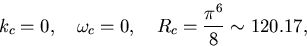(12)

 Neutral curve (1)